19
Jul 10

## R: Clash of the cannon cycles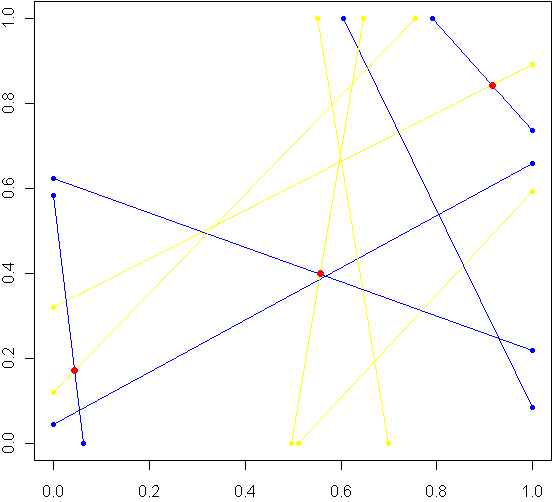Imagine a unit square. Every side has length 1, perfectly square. Now imagine this square was really a fence, and you picked two spots at random along the fence, with uniform probability over the length of the fence. At each of these two locations, set down a special kind of cannon. Just like the light cycles from Tron, these cannons leave trails of color. To aim each cannon, pick another random point from one of the three other sides of the fence, and aim for that point.

Sometimes there will be a collision within the square, other times no. The image at top shows the results of five trials. The red dots are where the trails from a pair of cannons collided. My burning question: What is the distribution for these dots? Before reading on, try to make a guess. Where will collisions be most likely to happen?

Somewhere in the world, there lives a probabilist who could come up with a formula for the exact distribution in an hour, but that person doesn’t live in my house, so I took the Monte Carlo approach, coded in R:

# Functions to pick two points, not on the same side:
m2pt <- function(m) {
if(m <1) {
myPoint = c(5,m,0)
} else if (m < 2) {
myPoint = c(6,1,m %% 1)
} else if (m < 3) {
myPoint = c(7,1-(m %% 1),1)
} else {
myPoint = c(8,0,1-(m %% 1))
}
return(myPoint)
}

get2pts <- function() {
pt1 = m2pt(runif(1,0,4))
pt2 = m2pt(runif(1,0,4))

# Make sure not both on the same sides. If so, keep trying
while(pt1 == pt2) {
pt2 = m2pt(runif(1,0,4))
}
return(c(pt1[2:3],pt2[2:3]))
}

# Optional plot of every cannon fire line. Not a good idea for "iters" more than 100
#windows()
#plot(0,0,xlim=c(0,1),ylim=c(0,1),col="white")

# How many times to run the experiment
iters = 10000

# Track where the intersections occur
interx = c()
intery = c()

for(i in 1:iters) {
can1 = get2pts()
can2 = get2pts()

# Optional plot of every cannon fire line. Not a good idea for "iters" more than 100
#points(c(can1,can1),c(can1,can1),pch=20,col="yellow")
#segments(can1,can1,can1,can1,pch=20,col="yellow",lwd=1.5)
#points(c(can2,can2),c(can2,can2),pch=20,col="blue")
#segments(can2,can2,can2,can2,pch=20,col="blue",lwd=1.5)

# See if there is a point of intersection, find it.
toSolve = matrix(c( can1-can1, can2-can2, can1-can1, can2-can2),byrow=T,ncol=2)
paras = solve(toSolve, c( can2-can1, can2-can1))

solution = c(can1 + paras*(can1-can1), can1 + paras*(can1-can1))

# Was the collision in the square
if(min(solution) > 0 && max(solution) < 1) {
# Optional plot of red dots
# points(solution,solution,pch=20,col="red",cex=1.5)

# if this intersection is in square, plot it, add it to list of intersections
interx = c(interx, solution)
intery = c(intery, solution)
}
}

windows()
plot(interx, intery, pch=20,col="blue",xlim=c(0,1),ylim=c(0,1))


After carefully writing and debugging much more code than I expected, I ran a trial with several thousand cannon fires and plotted just the collisions. Here is what I saw: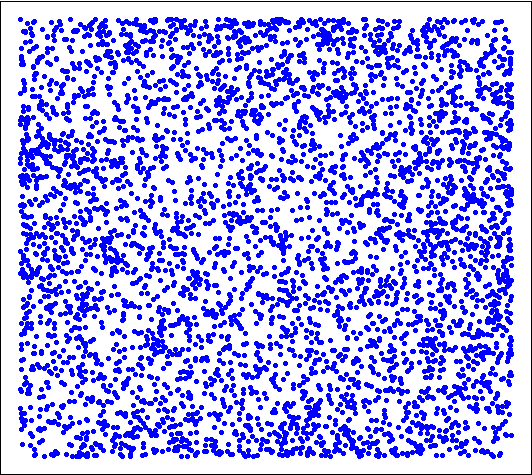Looks pretty uniform, doesn't it? If it is, I will have gone a very long way just to replicate the bi-variate uniform distribution. My own original guess was that most collisions, if they happened in the square, would be towards the middle. Clearly this wasn't the case. Looks can be deceiving, though, so I checked a histogram of the x's (no need to check the y's, by symmetry they have the same distribution):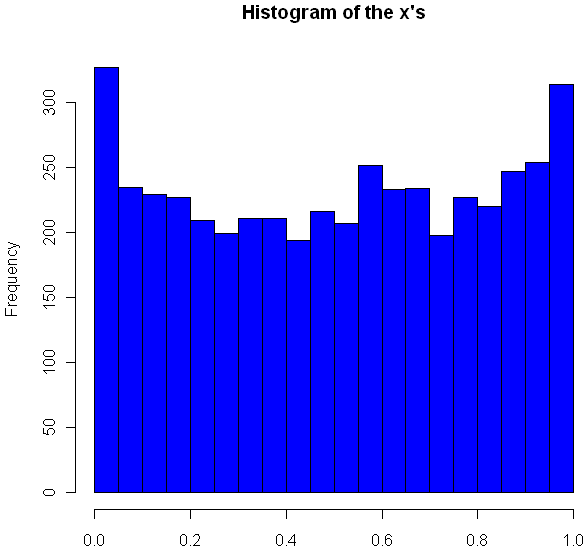Very interesting, no? The area near the edges appears more likely to have a collision, with an overall rounded bowl shape to the curve. The great thing about Monte Carlo simulations is that if something unexpected happens, you can always run it again with more trials. Here I changed "iters" to 100,000, ran the code again, and plotted the histogram.

hist(interx, breaks=100, col="blue",xlab="x",main="Histogram of the x's")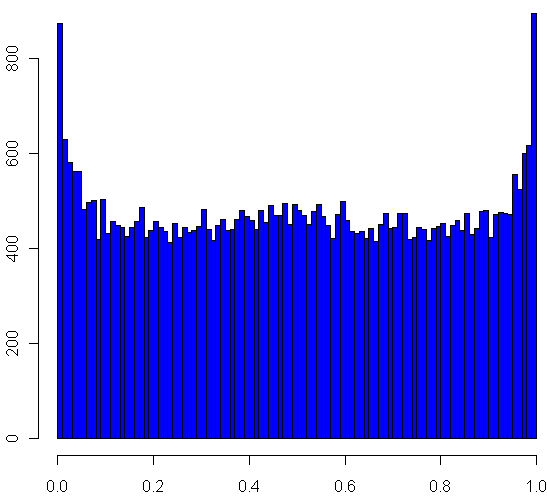Now its clear that the distribution spikes way up near the edges, and appears to be essentially flat for most of the middle area. It seems like it may even go up slightly at the very middle. Just to be sure, I ran a trial with one million iterations:

Now it definitely looks like a small upward bulge in the middle, though to be sure I would have to do run some statistical tests or use an even larger Monte Carlo sample, and given how inefficient my code is, that could take the better part of a week to run. So for today I'll leave it at that.

One final statistic of note: During my run of one million iterations, 47.22% of all collisions happened inside the box. What do you think, is the true, theoretical ratio of collisions within the box a rational number?

10
Jul 10

9
Jul 10

## 100 Prisoners, 100 lines of codeIn math and economics, there is a long, proud history of placing imaginary prisoners into nasty, complicated scenarios. We have, of course, the classic Prisoner’s Dilemma, as well as 100 prisoners and a light bulb. Add to that list the focus of this post, 100 prisoners and 100 boxes.

In this game, the warden places 100 numbers in 100 boxes, at random with equal probability that any number will be in any box. Each convict is assigned a number. One by one they enter the room with the boxes, and try to find their corresponding number. They can open up to 50 different boxes. Once they either find their number or fail, they move on to a different room and all of the boxes are returned to exactly how they were before the prisoner entered the room.

The prisoners can communicate with each other before the game begins, but as soon as it starts they have no way to signal to each other. The warden is requiring that all 100 prisoners find their numbers, otherwise she will force them to listen to hundreds of hours of non-stop, loud rock musician interviews. Can they avoid this fate?

The first thing you might notice is that if every prisoner opens 50 boxes at random, they will have a $0.5$ probability of finding their number. The chances that all of them will find their number is $(\frac{1}2)^{100}$, which is approximately as rare as finding a friendly alien with small eyes. Can they do better?

Of course they can. Otherwise I wouldn’t be asking the question, right? Before I explain how, and go into a Monte Carlo simulation in R, you might want to think about how they can do it. No Googling!

All set? Did you find a better way? The trick should be clear from the code below, but if not skip on to the explanation.

# How many times should we run this experiment?
iters = 1000
results = rep(0,iters)

for(i in 1:iters) {
# A random permutation:
boxes = sample(1:100,100)

# Labels for our prisoners
prisoners = 1:100

# Track how many "winners" we have
foundIt = 0

# Main loop over the prisoners
for(prisoner in prisoners) {

# Track the prisoners path
path = c(prisoner)

tries = 1

# Look first in the box that matches your own number
inBox = boxes[prisoner]

while(tries < 50) { 			 			path = c(path, inBox) 			 			if(inBox == prisoner) { 				#cat("Prisoner", prisoner, "found her number on try", tries, "\n") 				foundIt = foundIt + 1 				break; 			} else { 				# Follow that number to the next box 				inBox = boxes[inBox] 			} 			tries = tries+1 		} 		 		# cat("Prisoner", prisoner, "took path", paste(path, collapse=" -> "), "\n")
}

# How many prisoners found their numbers?
cat("A total of", foundIt, "prisoners found their numbers.\n")
flush.console()
results[i] = foundIt
}

hist(results, breaks=100, col="blue")


Here is what one of my plots looked like after running the code: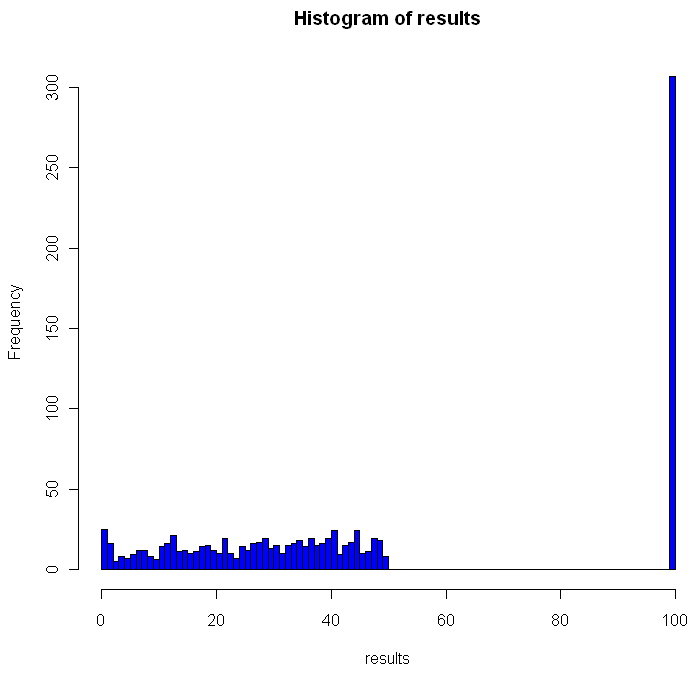Out of the 1000 times I ran the experiment, on 307 occasions every single prisoner found his number. The theoretical success rate is about 31%. So, if it’s not clear from the code, what was the strategy employed by the prisoners and how does it work?

One way to look at the distribution of numbers in boxes is to see it as a permutation of the numbers from 1 to 100. Each permutation can be partitioned into what are called cycles. A cycle works like this: pick any number in your permutation. Let’s say it’s 23. Then you look at the number the 23rd place (ie the number in the 23rd box, counting from the left). If that number is 16, you look at the number in the 16th place. If that number is 87, go open box number 87 and follow that number. Eventually, the box you open up will have the number that brings you back to where you started, completing the cycle. Different permutations have different cycles.

The key for the prisoner is that by starting with the box that is the same place from the left as his number, and by following the numbers in the boxes, the prisoner guarantees that if he is in a cycle of length less than 50, he will eventually open the box with his number in it, which would complete the cycle he began. One way to envision cycles of different lengths is to think about the extreme cases. If a particular permutation shifted every single number over one to the left (and wrapped number 1 onto the end), you would have a single cycle of length 100. Box 1 would contain number 2, box 2 number 3 and so on. On the other hand, if a permutation flipped every pair of consecutive numbers, you would have 50 cycles, each of length 2: box 1 would have number 2, box 2 would have number 1. Of course if your permutation doesn’t change anything you have 100 cycles of length 1.

As you can see from the histogram, when using this strategy you can never have between 50 and 100 winning prisoners. Anytime you have a single cycle of length greater than 50, for example 55, then all 55 prisoners who start on that cycle will fail to find their number. If no cycles are longer than 50, everyone wins. Just how rare are different cycles of different lengths? For the math behind that check out this excellent explanation by Peter Taylor of Queen’s University.

Before moving on I wanted to visualize these cycles. Try running the code below:

# Unit circle
plot(0,0,xlim=c(-1,1),ylim=c(-1,1),col="white",ann=FALSE, xaxt="n", yaxt="n")
for(i in 1:100) {
points(cos(2*i/100*pi), sin(2*i/100*pi),pch=20,col="gray")
}

mySample = sample(1:100,100)
for(i in 1:100) {
found = FALSE
nextItem = i

# Pick a random color for this cycle
color = sample(c(0:9,"A","B","C","D","E","F"),12,replace=T)
lineColor = paste("#", paste(color[1:6],collapse=""),sep="")

while(!found) {
# Draw the cycle

segments(cos(nextItem/50*pi), sin(nextItem/50*pi), cos(mySample[nextItem]/50*pi), sin(mySample[nextItem]/50*pi),col=lineColor,lwd=2)
Sys.sleep(.4)
if(mySample[nextItem] == i) {
found = TRUE
} else {
nextItem = mySample[nextItem]
}
}
}


You can adjust the “Sys.sleep()” parameter to make the animation faster. I recommend running the code to see how the cycles “develop” over time, but here’s a snapshot of what I got: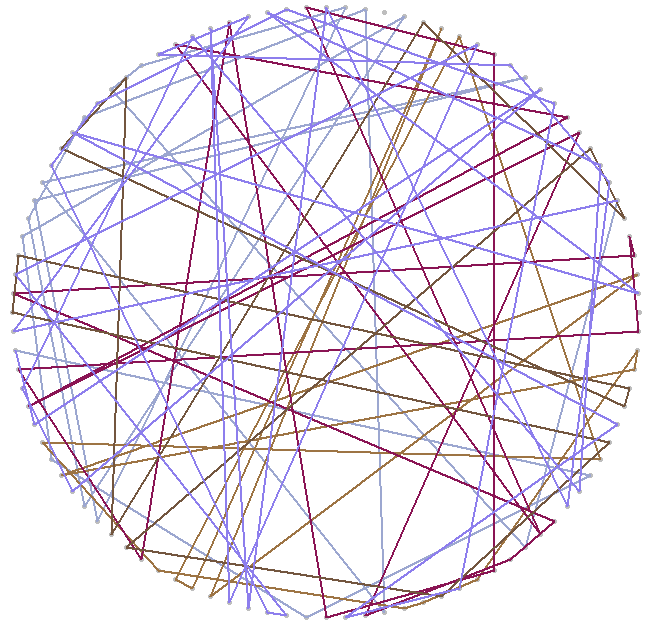2
Jul 10

## Word games in probability and R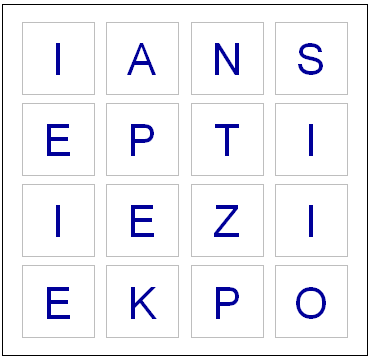Last night, while playing Boggle, we ended up with a board without a single vowel. Not even a “Y” or “Qu”. This seemed fairly unusual, so I wondered what the chances were of such an occurrence. I found an online list of the letters each die has, and I could have written down the number of vowels on each one by hand, but whenever possible I like to do things by computer. So I fired up Hal and asked for some help with the calculations.

Apparently some European boards use a 5 x 5 grid, but here in the land of the Maple leaf our board has 16 cubes. Here are the letters on them, as I coded them into R:

d1 = c('S','R','E','L','A','C')
d2 = c('D','P','A','C','E','M')
d3 = c('Qu','B','A','O','J','M')
d4 = c('D','U','T','O','K','N')
d5 = c('O','M','H ','R','S','A')
d6 = c('E','I','F','E','H','Y')
d7 = c('B','R','I','F','O','X')
d8 = c('R','L','U','W','I','G')
d9 = c('N','S','O','W','E','D')
d10 = c('Y ','L','I','B','A','T')
d11 = c('T','N','I','G','E','V')
d12 = c('T','A','C','I','T','O')
d13 = c('P','S','U','T','E','L')
d14 = c('E','P','I','S','H ','N')
d15 = c('Y','K','U','L','E','G')
d16 = c('N','Z','E','V','A','D')


So now I had to check how many vowels were on each die. Here’s the code I used for this:

vowels = c('A','E','I','O','U','Qu','y')
vowelsFound = rep(0,16)
for(i in 1:16) {
found = 0
die = eval(parse(text=paste("d",i,collapse="",sep="")))
for(l in die) {
# Check to see if this letter is in the vowels vector
if(l %in% vowels) {
found = found + 1
}
}
vowelsFound[i] = found
}

# Probabilities of getting a vowel for each die
pVowels = vowelsFound/6

# Probability of getting no vowel for each die
pNoVowels = 1 - pVowels

# Chance that we will get not a single vowel, including "y" and "Qu"
print(prod(pNoVowels))


If you run the code above, you should see that the probability of getting no vowels (including “Y” and “Qu”) is 0.000642. That works out to one in every 1557 boards. So it’s quite rare, but by no means so extraordinary that it crosses the Universal probability bound. Also, it’s not enough to just calculate how rare your event is, or how rare any similar or more extreme event is, and then be astounded. You also have to include all the other possible events that would have left you amazed. What about getting all vowels (much more rare)? What about getting 8 or 9 E’s, or a row or column of all A’s or E’s? It’s likely that if you add up all probabilities of all the rare events which might leave you amazed, you’ll end up with a good chance of amazement every time.

I could have stopped here, but having coded the dice, I decided to create a simplified version of the game in R. If I have a chance over the next few days I’ll add some more features.

# You will need to download a dictionary file. I found one here:
# http://svn.pietdepsi.com/repos/projects/zyzzyva/trunk/data/words/north-american/owl2-lwl.txt
words = read.table("wordlistData.dat", colClasses = "character")
words = unlist(words[,1])

# Create a random board. Plot it.
board = diag(4)
dice = sample(1:16,16)
cntr = 4
for(i in dice) {
die = eval(parse(text=paste("d",i,collapse="",sep="")))
board[floor(cntr/4), (cntr %% 4) + 1] = sample(die,1)
cntr = cntr + 1
}

plot(0,0,xlim=c(0,4),ylim=c(0,4),col="white",ann=FALSE, xaxt="n", yaxt="n" )

for(m in 1:4) {
for(n in 1:4) {
text(m-.5,n-.5,labels=board[m,n],cex=2.75,col="#000099")
# Draw a square the easy way
points(m-.5,n-.5,pch=0,cex=10,lwd=1.5,col="gray")
}
}

# How many seconds to give for each round
gameTime = 180

START_TIME = proc.time()
elapsed = 0

# Simple scoring, with 1 point per letter.
# Dictionary only has words length 3 or longer
score = 0

cat("Find words. Hit enter after each word.\n")
while(elapsed < gameTime) {
myWord = scan(n=1, what=character()) # Get a single word
elapsed = signif(proc.time() - START_TIME, digits=4)
if (length(myWord)==0) {
cat("You have", gameTime - elapsed, "seconds left. Keep going!\n")
} else {

if(elapsed < gameTime) {
# Check if it's a real word, see if it is in dictionary
# Convert their guess to uppercase letter
myWord = toupper(myWord)

# If it is in the dictionary, give them points
if(myWord %in% words) {
# Make sure they haven't used this word before TODO

# Add it to their score
score = score + nchar(myWord)
cat("Congratulations. You are up to", score, "points.")
cat("You have", gameTime - elapsed, "seconds left. Keep going!\n")
} else {
# If it isn't in the dictionary, let the user know that they got it wrong.
cat("Sorry, that is not in the dictionary. Keep trying!\n")
}

}
}
}

cat("Out of time! ")
cat("Your final score was:", score, "points.")


Enjoy the game. Let me know if you notice any issues or have suggestions!